• 拓扑排序是对有向无环图一种排序，满足如下两个条件： 1.每个顶点出现且只出现一次； 2.若A在序列中排在B前面，则在图中不存在从B到A路径。 如上无环有向图，v表示顶点：v=['a','b','c','d','e']，e表示...
   拓扑排序是对有向无环图的一种排序，满足如下两个条件：

1.每个顶点出现且只出现一次；

2.若A在序列中排在B的前面，则在图中不存在从B到A的路径。

如上的无环有向图，v表示顶点：v=['a','b','c','d','e']，e表示有向边：e=[('a','b'),('a','d'),('b','c'),('d','c'),('d','e'),('e','c')]，代码如下：

def indegree0(v,e):
if v==[]:
return None
tmp=v[:]
for i in e:
if i in tmp:
tmp.remove(i)
if tmp==[]:
return -1

for t in tmp:
for i in range(len(e)):
if t in e[i]:
e[i]='toDel' #占位，之后删掉
if e:
eset=set(e)
eset.remove('toDel')
e[:]=list(eset)
if v:
for t in tmp:
v.remove(t)
return tmp

def topoSort(v,e):
result=[]
while True:
nodes=indegree0(v,e)
if nodes==None:
break
if nodes==-1:
print('there\'s a circle.')
return None
result.extend(nodes)
return result

v=['a','b','c','d','e']
e=[('a','b'),('a','d'),('b','c'),('d','c'),('d','e'),('e','c')]
res=topoSort(v,e)
print(res)

indegree0函数返回入度为0的顶点，并在v和e中删除它和它相邻的边，如果v列表中没有顶点了，就返回None，如果v列表中还有顶点但是找不到入度为0的顶点，说明有向图中有环，返回-1。topoSort函数不断取出有向图中入度为0的顶点，最后就是拓扑排序序列。输出如下：
['a', 'b', 'd', 'e', 'c']

考虑一种有环的情况，加上一条c->d的边，如下图所示：

输出如下：

there's a circle.
None

参考文献：

https://blog.csdn.net/littlethunder/article/details/24113193
展开全文python
• 拓扑排序 几乎在所有项目，甚至日常生活，待完成不同任务之间通常都会存在着某些依赖...拓扑排序算法 任何无回路顶点活动网（AOV网）N都可以做出拓扑序列： 从N中选出一个入度为0顶点作为序列下一顶点。 从
• 又去学了迪杰斯特拉算法 其实和最下生成树prim基本差不多 就是遍历做标记然后循环，把最短记录下来，遇到更短就换掉 还有拓扑用数组容量不够了100000*100000 改用用链表解决了 把二维数组换成 一个结构体数组...
遇到floyd优化了也时间超限的题
只会floyd很难受
又去学了迪杰斯特拉算法
其实和最下生成树prim基本差不多
就是遍历做标记然后循环，把最短的记录下来，遇到更短的就换掉
还有拓扑用数组容量不够了100000*100000
改用用链表解决了
把二维数组换成 一个结构体数组然后再用指针不断地指子项这样的


展开全文• ## 拓扑排序算法

千次阅读 2013-11-26 20:58:13
拓扑排序算法是针对有向无环图（DAG）的一种排序方法，按照它的排序算法最终求得的序列中，一定满足如下条件：若属于e，e为图的边集，那么就有v0 在 v1的前面。 这样的序列称为拓扑序列，...那么拓扑排序的步骤是： 1）
拓扑排序算法是针对有向无环图（DAG）的一种排序方法，按照它的排序算法最终求得的序列中，一定满足如下条件：若<v0,v1>属于e，e为图的边集，那么就有v0 在 v1的前面。
这样的序列称为拓扑序列，拓扑排序广泛用于一些具有明显优先级的问题中，最典型的应用就是先修和后修课程，如和安排课程，不发生矛盾。注意：拓扑排序算法的基本要求是图汇总不存在环，原因很明显，如果有环就会产生矛盾。那么拓扑排序的步骤是：
1）先将图中每个顶点的入度求出来，保存在数组中
2）利用栈或队列将入度为零的顶点保存
3）设置一个记录循环个数的变量count
4）出栈（任意一个入度为零的顶点），然后将与其相邻的顶点的入度减一，（在图中表示为：将相应的边给除掉），判断减一后的顶点的入度是否为0，若为零则入栈或队列。
5)不断循环步骤4，直至栈或队列为空。
6）判断count与图中顶点个数的大小，若count值小于图中顶点个数，那么就说明图中存在环，不存在拓扑序列。否则存在拓扑序列。
算法结束。
下面是代码：
//图的邻接矩阵表示法
#include <stdio.h>
#include <stdlib.h>
#include <string.h>
#include <stack>
#include <queue>
using namespace std;
#define Max 100
#define Inf 0x1111
typedef char type;
typedef struct Grap{
type data[Max];
int value[Max][Max];
int n,m;
}Grap,*pgrap;
int Located(pgrap g,char ch){
for(int i=0;i<g->n;i++)
if(g->data[i]==ch)
return i;
}
int invalue[Max];
void Creat_grap(pgrap g){
printf("输入图的顶点数和边数:\n");
scanf("%d%d",&g->n,&g->m);
//printf("ksgfdkj\n");
getchar();
printf("输入图中的顶点:\n");
int i,j;
for(i=0;i<g->n;i++){
g->data[i]=getchar();
getchar();
}
for(i=0;i<g->n;i++)
for(j=0;j<g->n;j++)
g->value[i][j]=Inf;
printf("请输入图中的边:\n");
int index1,index2,value;
char ch1,ch2;
while(g->m--){
scanf("%c,%c,%d",&ch1,&ch2,&value);
getchar();
index1=Located(g,ch1);
index2=Located(g,ch2);
g->value[index1][index2]=value;
//无向图
//g->value[index2][index1]=value;
}
}
void In_value(pgrap g){
memset(invalue,0,sizeof(invalue));
for(int i=0;i<g->n;i++)
for(int j=0;j<g->n;j++)
if(g->value[j][i]!=Inf)
invalue[i]++;
}
void tuopu(pgrap g){
int i;
stack<int> s;
//queue<int> q;
for(i=0;i<g->n;i++)
if(invalue[i]==0)
s.push(i);
int index,count=0;
while(!s.empty()){
index=s.top();
printf("%c ",g->data[index]);
//q.push(index);
s.pop();
count++;
for(i=0;i<g->n;i++)
if(g->value[index][i]!=Inf)
if(--invalue[i]==0)
s.push(i);
}
if(count<g->n)
printf("存在环\n");
}
int main(){
Grap g;
pgrap p=&g;
Creat_grap(p);
In_value(p);
tuopu(p);
return 0;
}


下面是测试数据：

输入图的顶点数和边数:
6 9
输入图中的顶点:
A
B
C
D
E
F
请输入图中的边:
A,B,1
A,C,3
B,D,4
B,C,1
C,D,1
D,E,2
C,E,1
E,F,1
D,F,1
A B C D E F
Terminated with return code 0
Press any key to continue ...
下面是用邻接表是实现的拓扑排序代码：
//图的存储结构
//邻接表
#include <stdio.h>
#include <stdlib.h>
#include <queue>
#include <stack>
#include <string.h>
using namespace std;
#define Max 100 //顶点个数最多为100个
typedef char type;
typedef struct Arcnode{
int index;
int value;
struct Arcnode *next;
}Arcnode,*parc;
typedef struct Node{
type data;
Arcnode *first;
}Node;
typedef struct Grap{
Node nodetex[Max];
int n; //顶点数
int m; //边数
}Grap,*pgrap;
int invalue[Max];
int Located(pgrap g,char ch){
for(int i=0;i<g->n;i++)
if(ch==g->nodetex[i].data)
return i;
}
void Creat_grap(pgrap g){
printf("输入图的结点数和边数:\n");
scanf("%d%d",&g->n,&g->m);
getchar();
int i,index1,index2,value;
char ch1,ch2;
printf("输入各顶点:\n");
for(i=0;i<g->n;i++){
g->nodetex[i].data=getchar();
g->nodetex[i].first=NULL;
getchar();
}
printf("输入各边及权值:\n");
parc q;
for(i=0;i<g->m;i++){
scanf("%c,%c,%d",&ch1,&ch2,&value);
getchar();
index1=Located(g,ch1);
index2=Located(g,ch2);
q=(parc)malloc(sizeof(Arcnode));
q->index=index2;
q->value=value;
q->next=g->nodetex[index1].first;
g->nodetex[index1].first=q;
//无向图(默认为任意两个顶点之间之多有一条边且自身到自身无边）
/*q=(parc)malloc(sizeof(Arcnode));
q->index=index1;
q->value=value;
q->next=g->nodetex[index2].first;
g->nodetex[index2].first=q;*/
}
}

void Delete_grap(pgrap g){
parc p,q;
for(int i=0;i<g->n;i++){
p=g->nodetex[i].first;
while(p){
q=p->next;
free(p);
p=q;
}
}
}
void In_value(pgrap g){
memset(invalue,0,sizeof(invalue));
for(int i=0;i<g->n;i++){
parc p=g->nodetex[i].first;
while(p){
invalue[p->index]++;
p=p->next;
}
}
}
void tuopu(pgrap g){
stack<int> s;
//queue<int> q;
int i,index,count=0;
for(i=0;i<g->n;i++)
if(invalue[i]==0)
s.push(i);
while(!s.empty()){
index=s.top();
printf("%c ",g->nodetex[index].data);
s.pop();
//q.push(index);
count++;
parc p=g->nodetex[index].first;
while(p){
if(--invalue[p->index]==0)
s.push(p->index);
p=p->next;
}
}
if(count<g->n)
printf("存在环\n");
}

int main(){
Grap g;
pgrap p=&g;
Creat_grap(p);
In_value(p);
tuopu(p);
Delete_grap(p);
return 0;
}

测试数据：
输入图的结点数和边数:
6 9
输入各顶点:
A
B
C
D
E
F
输入各边及权值:
A,B,1
A,C,3
B,D,4
B,C,1
C,D,1
D,E,2
C,E,1
E,F,1
D,F,1
A B C D E F
Terminated with return code 0
Press any key to continue ...


展开全文• 目录拓扑排序实现逻辑逆拓扑排序算法一（类似拓扑排序）：算法二（深度搜索）： 拓扑排序 实现逻辑 用邻接表表示图 #include <iostream> #include <stack> #include <queue> #include <...
目录拓扑排序实现逻辑逆拓扑排序算法一（类似拓扑排序）：算法二（深度搜索）：
拓扑排序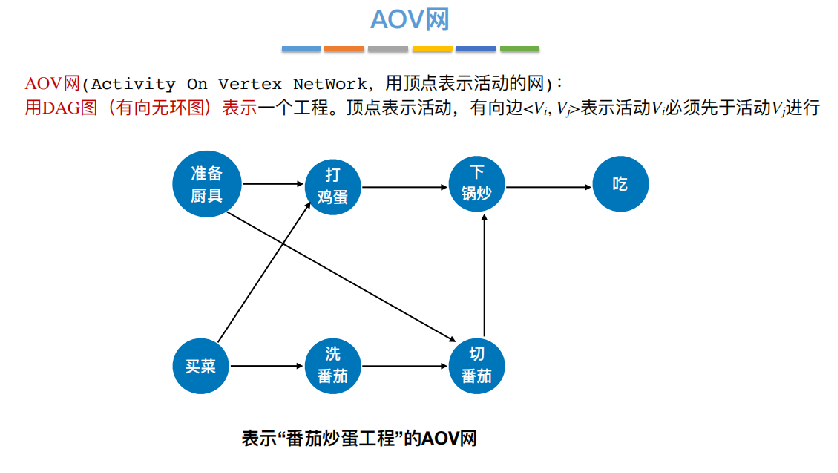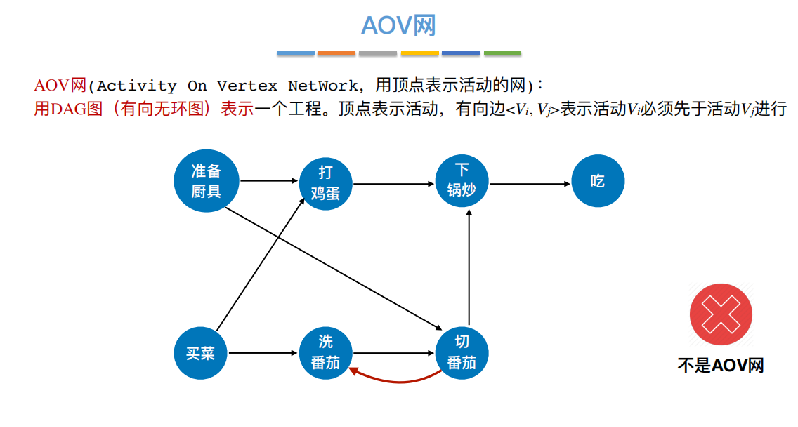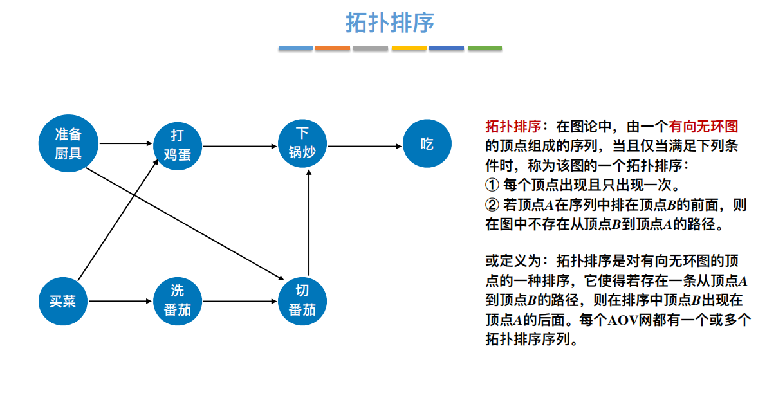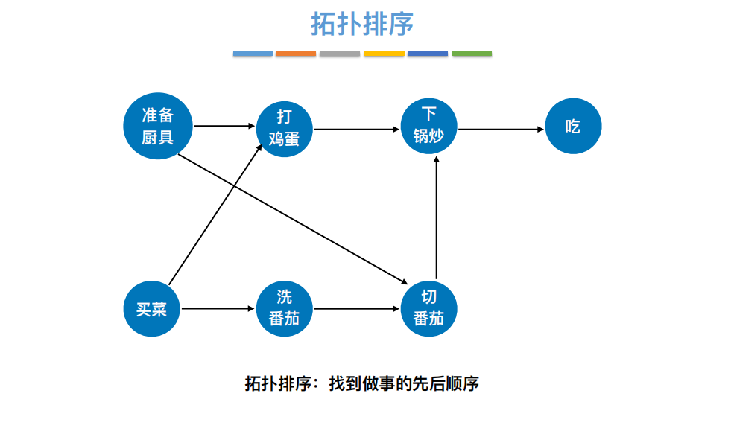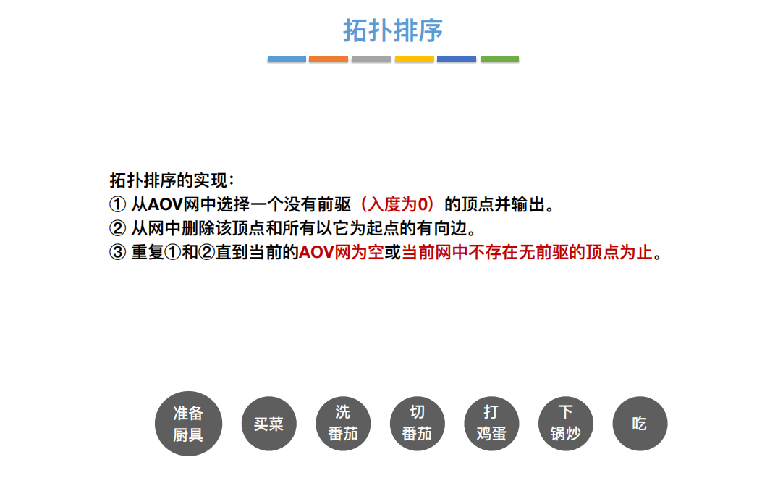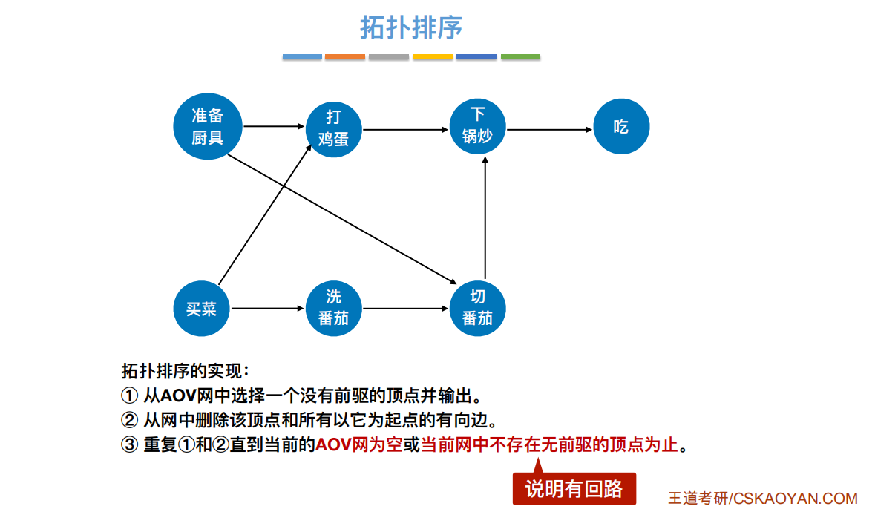实现逻辑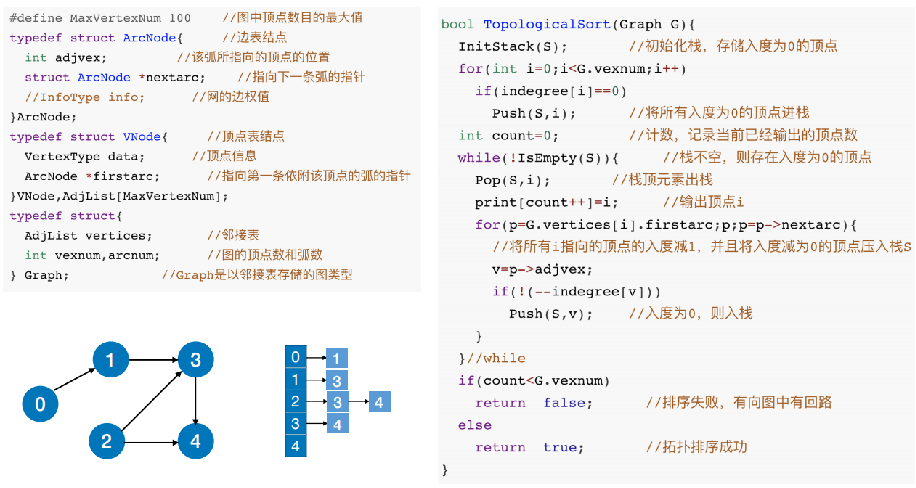用邻接表表示图
#include <iostream>
#include <stack>
#include <queue>
#include <vector>
#include <vector>
#include <algorithm>
#include <string.h>
using namespace std;
const int MaxVertexNum=10;
struct edgeListNode
{
int adjId;
int weight;
edgeListNode* nextedge=nullptr;
};
struct vertexListNode
{
int data;
edgeListNode* firstedge=nullptr;
};
struct Graph
{
int vertexnum=0;
int edgenum=0;
vertexListNode vertexList[MaxVertexNum];
};
void InsertEdge(Graph& graph,int i,int j)
{
edgeListNode* edge=new edgeListNode;
edge->adjId=j;
edge->nextedge=graph.vertexList[i].firstedge;//头插
graph.vertexList[i].firstedge=edge;
}
void GraphInitiation(Graph& graph)
{
graph.vertexnum=5;
graph.edgenum=5;
for(int i=0;i<graph.vertexnum;i++)//顶点表初始化
graph.vertexList[i].data=i;
vector<vector<int>>M={{0,1},{1,3},{2,3},{2,4},{3,4}};
for(int i=0;i<M.size();i++)//边表初始化
InsertEdge(graph,M[i],M[i]);
for(int i=0;i<graph.vertexnum;i++)
{
printf("%d",graph.vertexList[i].data);
edgeListNode* edge=graph.vertexList[i].firstedge;
if(edge)
printf("-->");
while(edge)
{
printf("%d",graph.vertexList[edge->adjId]);
edge=edge->nextedge;
if(edge)
printf("-->");
}
printf("\n");
}
}

拓扑排序
unsigned int CalIndegree(Graph& graph,int v)
{
uint cnt=0;
for(int i=0;i<graph.vertexnum && i!=v;i++)
{
edgeListNode* edge=graph.vertexList[i].firstedge;
while(edge)
{
if(edge->adjId==v)
cnt++;
edge=edge->nextedge;
}
}
return cnt;
}
bool ToPoSort(Graph& graph)
{
queue<int>Q;
uint Indegree[graph.vertexnum];
for(int i=0;i<graph.vertexnum;i++)
if(!(Indegree[i]=CalIndegree(graph,i)))
Q.push(i);
uint cnt=0;
while(!Q.empty())
{
int k=Q.front();
Q.pop();
cnt++;
printf("%d ",k);
for(edgeListNode* edge=graph.vertexList[k].firstedge;edge;edge=edge->nextedge)
{
int v=edge->adjId;
if(!(Indegree[v]=Indegree[v]-1))
Q.push(v);
}
}
if(cnt<graph.vertexnum)
return 0;
else
return 1;
}

测试
int main()
{
Graph graph;
GraphInitiation(graph);
printf("拓扑排序是：");
ToPoSort(graph);
printf("\n");
}

运行结果
0-->1
1-->3
2-->4-->3
3-->4
4
拓扑排序是：0 2 1 3 4

逆拓扑排序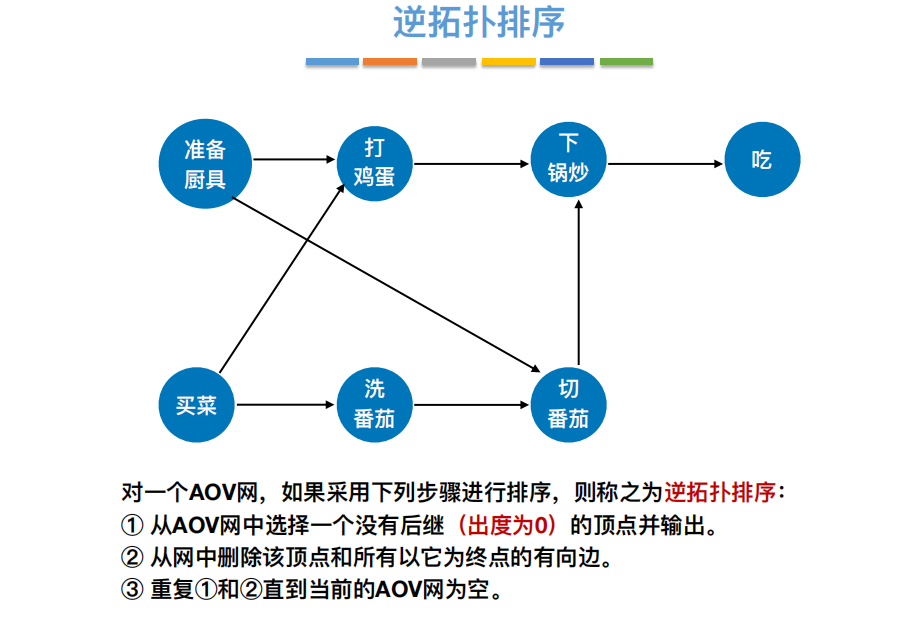算法一（类似拓扑排序）：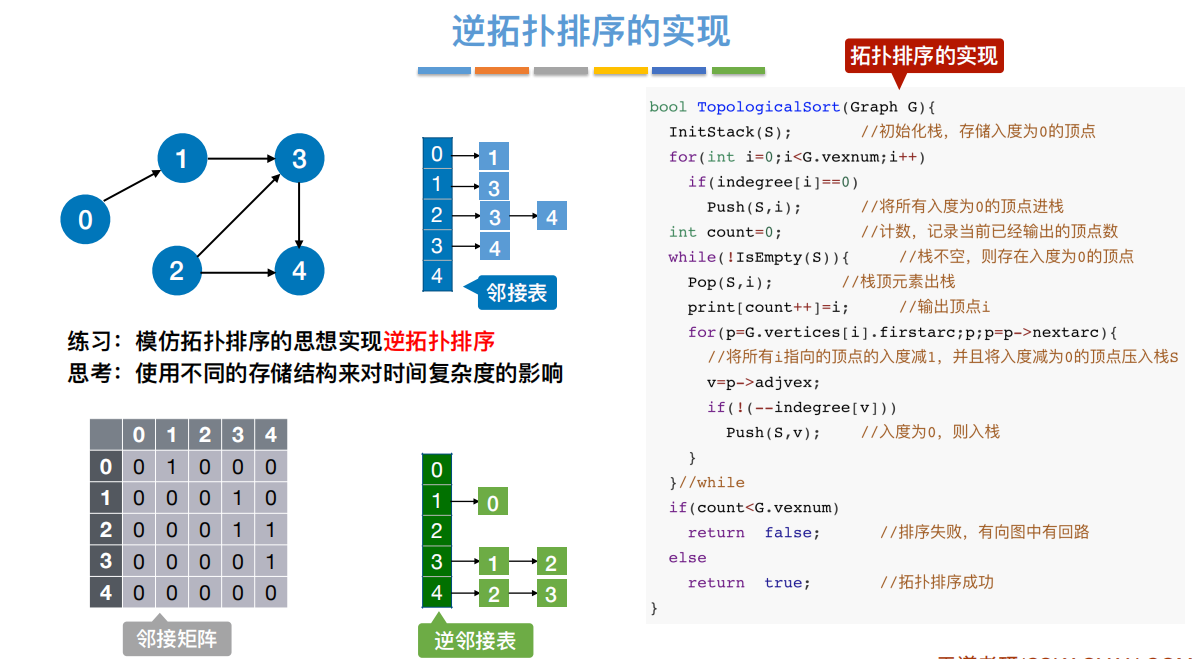代码
unsigned int CalOutdegree(Graph& graph,int v)
{
uint cnt=0;
edgeListNode* edge=graph.vertexList[v].firstedge;
while(edge)
{
cnt++;
edge=edge->nextedge;
}
return cnt;
}
bool ToPoSort(Graph& graph)
{
queue<int>Q;
uint Outdegree[graph.vertexnum];
for(int i=0;i<graph.vertexnum;i++)
if(!(Outdegree[i]=CalOutdegree(graph,i)))
Q.push(i);
uint cnt=0;
while(!Q.empty())
{
int k=Q.front();
Q.pop();
cnt++;
printf("%d ",k);
for(int i=0;i<graph.vertexnum && i!=k;i++)
{
edgeListNode* edge=graph.vertexList[i].firstedge;
while(edge)
{
if(edge->adjId==k)
{
Outdegree[i]=Outdegree[i]-1;
if(!Outdegree[i])
Q.push(i);
break;
}
edge=edge->nextedge;
}
}
}
if(cnt<graph.vertexnum)
return 0;
else
return 1;
}
int main()
{
Graph graph;
GraphInitiation(graph);
printf("逆拓扑排序是：");
ToPoSort(graph);
printf("\n");
}

运行结果
0-->1
1-->3
2-->4-->3
3-->4
4
逆拓扑排序是：4 3 1 2 0

算法二（深度搜索）：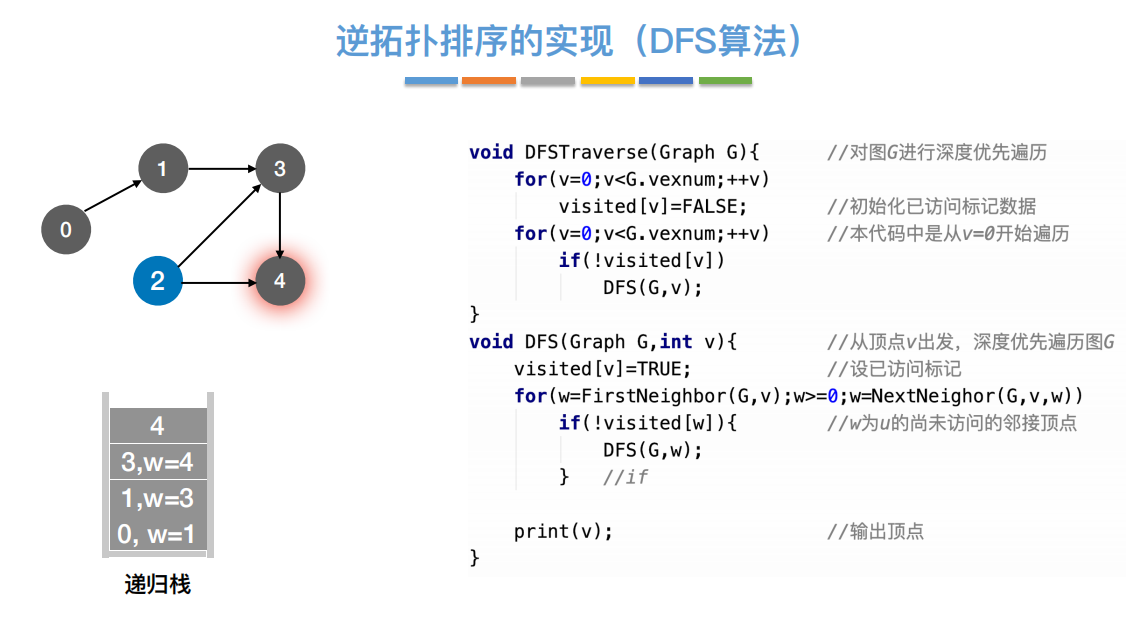void dfs(Graph& graph,bool* visited,int v)
{
visited[v]=true;
edgeListNode* edge=graph.vertexList[v].firstedge;
if(edge)
{
if(!visited[edge->adjId])
dfs(graph,visited,edge->adjId);
edge=edge->nextedge;
}
printf("%d ",v);
}
void ToPoSort_DFS(Graph& graph,bool* visited)
{
for(int i=0;i<graph.vertexnum;i++)
if(!visited[i])
dfs(graph,visited,i);
}
int main()
{
Graph graph;
GraphInitiation(graph);
bool visited[graph.vertexnum];
memset(visited,0,graph.vertexnum);
printf("逆拓扑排序为：");
ToPoSort_DFS(graph,visited);
printf("\n");
}

运行结果
0-->1
1-->3
2-->4-->3
3-->4
4
逆拓扑排序为：4 3 1 0 2



展开全文c++
• 图——基本的图算法（三）拓扑排序 1. 基本概念 对于一个有向无环图G = (V, E)来说，其拓扑排序就是G中所有顶点的一种线性排序，这种排序满足如下条件：如果图G中包含边(a...对一个有向无环图进行拓扑排序的基本思路...
• 定义：对有向无回路图G=(V,E)进行须拓扑排序后，结果为该图所有顶点一个线性序列，满足如果G包含边(u, v)，则在该序列中，u就出现在v前面（如果图是有回路，就不可能存在这样线性序列）。 定理：一个有向图...
• 要知道，如果你要用c++打出拓扑排序的代码，你先要学会c++语法和拓扑排序的原理，你要学会c++语法你先要学会基本数学和计算机基础知识（←Are U sure？），你要学会拓扑排序的原理，你先要学会计算机基础知识和栈的...
•  采用DFS我们可以给有向无环图进行拓扑排序，生成一个该图顶点线性序列，满足对于边(u,v)，在该序列中，u出现在v前面，如果有回路，那么就不会生成这样序列。  我们可以利用DFS后，各定点f域，即结束...深度优先搜索 DFS C++
• 有向图基本算法 对于有向图结构，和无向图类似，甚至更简单，有疑问请留言。 1>有向图数据结构 package graphTheory; /** * @author : 罗正 * @date time：2016年9月19日 下午9:26:21 **/ public class ...Kosaraju
• 理解拓扑排序，首先要大致了解一下拓扑序列，设G=(V，E)是一个具有n个顶点有向图，V中顶点序列v1, v2, …, vn称为一个拓扑序列，那么这个序列当且仅当满足下列条件：若从顶点vi到vj有一条路径，则在顶点序列中...
• 　在大学数据结构课上，我们知道求拓扑排序的一种方法。首先用一个入度数组保存每个顶点的入度。在进行拓扑排序时，我们需要找到入度为0的点，将其存入线性序列中，再将其从图中删除（与它相关的边都删除，相邻的...
• 　在大学数据结构课上，我们知道求拓扑排序的一种方法。首先用一个入度数组保存每个顶点的入度。在进行拓扑排序时，我们需要找到入度为0的点，将其存入线性序列中，再将其从图中删除（与它相关的边都删除，相邻的...
• 的拓扑排序内容比较简单，我们只要知道AOV网是一种以顶点表示活动、以边表示活动先后顺序且没有回路有向图即可。
• 拓扑排序算法基本思想: 1、从有向图中选取一个没有前驱（入度为0）顶点，并输出之 2、从有向图中删去此顶点以及所有以它为尾弧 3、重复上述两步，直至图空，或者图不空但找不到无前驱顶点为止 数据...
• 的基本算法-拓扑序列拓扑排序拓扑排序基本算法代码示例 拓扑排序 对于一个有向无环图，简称DAG（Directed Acyclic Graph），进行拓扑排序就是将图中所有的定点排成一个线性序列，使得图中任意一对定点u和v，若边&...数据结构
• 在一个表示工程的有向图中，用顶点表示活动，用弧表示... 对AOV网进行拓扑排序的基本思路是：从AOV网中选择一个入度为0的顶点输出，然后删去此顶点，并删除以此顶点为尾的弧，继续重复此步骤，直到输出全部顶点或...
• 对AOV网进行拓扑排序的基本思路是:从AOV网中选择一个入度为0的顶点输出，然后删去此顶点，并删除以此顶点为尾的弧，继续重复此步骤，直到输出全部顶点或者AOV 网中不存在入度为0的顶点为止。 首先我们需要确定一下这...数据结构
• 河南工程学院数据结构与算法课程设计 成果报告 拓扑排序算法实现 学生学号 学生姓名 学 院 计算机学院 专业班级 软件工程1342 专业课程 数据结构与算法 指导教师 2014 年 12 月 29 日 题 目 拓扑排序算法实现 考核...
• 关于拓扑排序的概念及基本思想，我在上一篇文章中已经较为详细的描述了，这里不在介绍。我们知道利用邻接矩阵进行拓扑排序时，程序实现较为简单，但是效率不高，算法的复杂度为O(n^3).而利用邻接表会使入度为0的顶点......